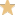×
Log in to StudySoup
Get Full Access to UNT - CSCE 4110 - Class Notes - Week 1
Join StudySoup for FREE
Get Full Access to UNT - CSCE 4110 - Class Notes - Week 1

Already have an account? Login here
×
Reset your password

UNT / Education and Teacher Studies / TEA 4110 / What is Fibonacci Sequence?

# What is Fibonacci Sequence? Description

##### Uploaded: 01/23/2017
2 Pages 91 Views 8 Unlocks
Reviews

## How many recursions is this?## What makes up a good algorithm?## What is an algorithm?Week 1 Tuesday,  January 17, 2017 2:22 PMCourse website: http://cse.unt.edu/~4110s001/ What is an algorithm? • A set of finite instructions What makes up a good algorithm? • Running time is a polynomial of the input. (exponential vs. polynomial) • Fibonacci Sequence (Bad Algorithm) Code: fibo(N) ○ If (N=0) or (N=1) then fibo <-- N Else fibo <-- fibo(N-1) + fibo(N) Bad functiDon't forget about the age old question of georgia tech linear algebra 1554
Don't forget about the age old question of Who developed the hierarchy of needs?
Don't forget about the age old question of What are the sub disciplines of Anatomy?
We also discuss several other topics like ud winter session
Don't forget about the age old question of opsy medical term
Don't forget about the age old question of What is meant by Margin of Error?
on because (2N ○ ) How many recursions is this? ○ ▪ Depicted as a tree. ▪ Left branch has a depth of N ▪ Right branch has a depth of N/2 For a complete tree 2N/2  ▪ recursions Computers Grew Out of Multiple Disciplines • Math - Numerical Analysis • Biology - Trees and Hierarchies • Psychology - Clique (subgraphs/graph theory) • Linguistics - compilers (Chomsky's hierarchy) Recurrence Notation: T(n) Sorting Insertion Sort ϴ(n2) Bubble Sort  ϴ(n2) T(n) = i=n∑1i  = i=1∑n • i = n(n+1)/2 • The above formula is known as the Arithmetic Sum. Merge Sort ϴ(nlogn) • Power of divide and conquer • T(n) = 2t(n/2) + n, n>1, T(1) = 1 Assume : n = 2k ○ ○ T(n) = 2[2T(n/2) +n/2] + n         = 22 ○ T(n/4) + n + n         = 22 ○ T(n/4) +  2n   =3 • T(n) = 2t(n/2) + n, n>1, T(1) = 1 Assume : n = 2k ○ ○ T(n) = 2[2T(n/2) +n/2] + n         = 22 ○ T(n/4) + n + n         = 22 ○ T(n/4) +  2n           = 23 ○ T(n/8) +  3n         = 2kT(n/2k ○ ) + kn         = 2k ○ + kn ○         = n + kn ○         = n + nlogn ○         = ϴ(nlogn) • Ideally at the end of merge sort you would use Insertion Sort to fully use the  Divide and Conquer Paradigm. • Why would someone not just split merge sort in 3 rather than 2, to speed up  sort time? ○ Solution to the recurrence relation found on practice  sheet (problem #6) Division by 2 is a simple shift and uses less merges while division by 3 is no  ○ longer trivial programmatically and uses more merges.  Prim's Algorithm • ϴ(mlogn) given a priority queue data structure ϴ(n2 • ) given a array data structure • Minimal Spanning Tree Solution Kruskal's Algorithm • Minimal Spanning Tree  solution Elementary Algorithms • Depth First Sort • Topological SortThe first assignment will be assigned next week.

Page ExpiredIt looks like your free minutes have expired! Lucky for you we have all the content you need, just sign up here
References: